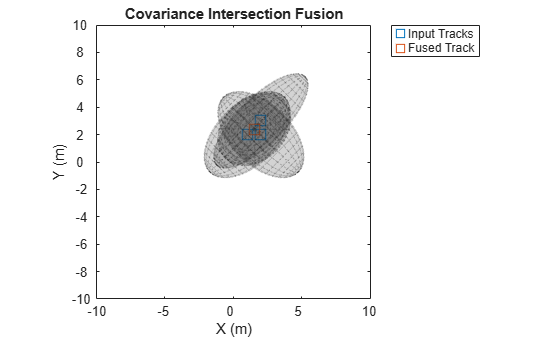# fusecovint

Covariance fusion using covariance intersection

## Syntax

``[fusedState,fusedCov] = fusecovint(trackState,trackCov)``
``[fusedState,fusedCov] = fusecovint(trackState,trackCov,minProp)``

## Description

example

````[fusedState,fusedCov] = fusecovint(trackState,trackCov)` fuses the track states in `trackState` and their corresponding covariance matrices `trackCov`. The function computes the fused state and covariance as an intersection of the individual covariances. It creates a convex combination of the covariances and finds weights that minimize the determinant of the fused covariance matrix.```

example

````[fusedState,fusedCov] = fusecovint(trackState,trackCov,minProp)` estimates the fused covariance by minimizing `minProp`, which can be either the determinant or the trace of the fused covariance matrix.```

## Examples

collapse all

Define a state vector of tracks.

```x(:,1) = [1;2;0]; x(:,2) = [2;2;0]; x(:,3) = [2;3;0];```

Define the covariance matrices of the tracks.

```p(:,:,1) = [10 5 0; 5 10 0; 0 0 1]; p(:,:,2) = [10 -5 0; -5 10 0; 0 0 1]; p(:,:,3) = [12 9 0; 9 12 0; 0 0 1]; ```

Estimate the fused state vector and its covariance.

`[fusedState,fusedCov] = fusecovint(x,p);`

Use `trackPlotter` to plot the results.

```tPlotter = theaterPlot('XLim',[-10 10],'YLim',[-10 10],'ZLim',[-10 10]); tPlotter1 = trackPlotter(tPlotter, ... 'DisplayName','Input Tracks','MarkerEdgeColor',[0.000 0.447 0.741]); tPlotter2 = trackPlotter(tPlotter,'DisplayName', ... 'Fused Track','MarkerEdgeColor',[0.850 0.325 0.098]); plotTrack(tPlotter1,x',p) plotTrack(tPlotter2,fusedState',fusedCov) title('Covariance Intersection Fusion')```Define a state vector of tracks.

```x(:,1) = [1;2;0]; x(:,2) = [2;2;0]; x(:,3) = [2;3;0];```

Define the covariance matrices of the tracks.

```p(:,:,1) = [10 5 0; 5 10 0; 0 0 1]; p(:,:,2) = [10 -5 0; -5 10 0; 0 0 1]; p(:,:,3) = [12 9 0; 9 12 0; 0 0 1]; ```

Estimate the fused state vector and its covariance. Combine the original covariances so that the trace of the fused covariance matrix is minimized.

`[fusedState,fusedCov] = fusecovint(x,p,'trace');`

Use `trackPlotter` to plot the results.

```tPlotter = theaterPlot('XLim',[-10 10],'YLim',[-10 10],'ZLim',[-10 10]); tPlotter1 = trackPlotter(tPlotter, ... 'DisplayName','Input Tracks','MarkerEdgeColor',[0.000 0.447 0.741]); tPlotter2 = trackPlotter(tPlotter, ... 'DisplayName','Fused Track','MarkerEdgeColor',[0.850 0.325 0.098]); plotTrack(tPlotter1,x',p) plotTrack(tPlotter2,fusedState',fusedCov) title('Covariance Intersection Fusion')```## Input Arguments

collapse all

Track states, specified as an N-by-M matrix, where N is the dimension of the state and M is the number of tracks.

Data Types: `single` | `double`

Track covariance matrices, specified as an N-by-N-by-M array, where N is the dimension of the state and M is the number of tracks.

Data Types: `single` | `double`

Property to minimize when estimating the fused covariance, specified as `'det'` or `'trace'`.

.

Data Types: `char` | `string`

## Output Arguments

collapse all

Fused state, returned as an N-by-1 vector, where N is the dimension of the state.

Fused covariance matrix, returned as an N-by-N matrix, where N is the dimension of the state.

 Matzka, Stephan, and Richard Altendorfer. "A comparison of track-to-track fusion algorithms for automotive sensor fusion." In Multisensor Fusion and Integration for Intelligent Systems, pp. 69-81. Springer, Berlin, Heidelberg, 2009.

 Julier, Simon, and Jeffrey K. Uhlmann. "General decentralized data fusion with covariance intersection." In Handbook of multisensor data fusion, pp. 339-364. CRC Press, 2017.